Courses

# Binomial Expansion - Interpolation and Extrapolation, Business Mathematics and Statistics B Com Notes | EduRev

Created by: Arshit Thakur

## B Com : Binomial Expansion - Interpolation and Extrapolation, Business Mathematics and Statistics B Com Notes | EduRev

The document Binomial Expansion - Interpolation and Extrapolation, Business Mathematics and Statistics B Com Notes | EduRev is a part of the B Com Course Business Mathematics and Statistics.
All you need of B Com at this link: B Com

The Binomial Theorem is a quick way (okay, it's a less slow way) of expanding (or multiplying out) a binomial expression that has been raised to some (generally inconveniently large) power. For instance, the expression (3x – 2)10 would be very painful to multiply out by hand. Thankfully, somebody figured out a formula for this expansion, and we can plug the binomial 3x – 2and the power 10 into that formula to get that expanded (multiplied-out) form.

The formal expression of the Binomial Theorem is as follows: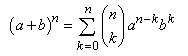Yeah, I know; that formula never helped me much, either. And it doesn't help that different texts use different notations to mean the same thing. The parenthetical bit above has these equivalents: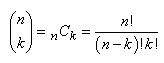Recall that the factorial notation "n!" means " the product of all the whole numbers between 1 and n", so, for instance, 6! = 1×2×3×4×5×6. Then the notation "10C7" (often pronounced as "ten, choose seven") means: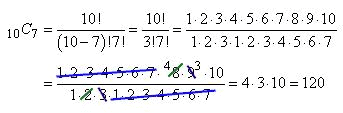Many calculators can evaluate this "n choose m" notation for you. Just look for a key that looks like "nCm" or "nCr", or for a similar item on the "Prob" or "Math" menu, or check your owner's manual under "probability" or "combinations".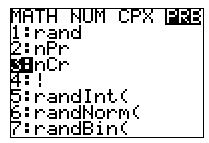The evaluation will probably look something like this: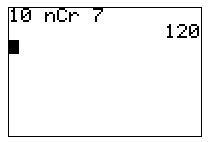There is another way to find the value of "nCr", and it's called "Pascal's Triangle". To make the triangle, you start with a pyramid of three 1's, like this: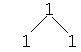Then you get the next row of numbers by adding the pairs of numbers from above. (Where there is only one number above, you just carry down the 1.)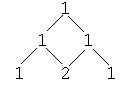Keep going, always adding pairs of numbers from the previous row..

To find, say, 6C4, you go down to the row where there is a "6" after the initial "1", and then go over to the 5th (not the 4th) entry, to find that 6C4 = 15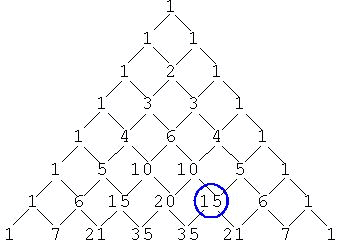As you might imagine, drawing Pascal's Triangle every time you have to expand a binomial would be a rather long process, especially if the binomial has a large exponent on it. People have done a lot of studies on Pascal's Triangle, but in practical terms, it's probably best to just use your calculator to find nCr, rather than using the Triangle. The Triangle is cute, I suppose, but it's not terribly helpful in this context, being more time-consuming than anything else. For instance, on a test, do you want to evaluate "10C7" by calculating eleven rows of the Triangle, or by pushing four buttons on your calculator?

I could never remember the formula for the Binomial Theorem, so instead, I just learned how it worked. I noticed that the powers on each term in the expansion always added up to whatever n was, and that the terms counted up from zero to n. Returning to our intial example of (3x – 2)10, the powers on every term of the expansion will add up to 10, and the powers on the terms will increment by counting up from zero to 10:

(3x – 2)10 = 10C0 (3x)10–0(–2)0 + 10C1 (3x)10–1(–2)1 + 10C2 (3x)10–2(–2)2

10C3 (3x)10–3(–2)3 + 10C4 (3x)10–4(–2)4 + 10C5 (3x)10–5(–2)5

10C6 (3x)10–6(–2)6 + 10C7 (3x)10–7(–2)7 + 10C8 (3x)10–8(–2)8

10C9 (3x)10–9(–2)9 + 10C10 (3x)10–10(–2)10

Note how the highlighted counter number counts up from zero to 10, with the factors on the ends of each term having the counter number, and the factor in the middle having the counter number subtracted from 10. This pattern is all you really need to know about the Binomial Theorem; this pattern is how it works.

Your first step, given a binomial to expand, should be to plug it into the Theorem, just like I did above. Don't try to do too many steps at once. Only after you've set up your binomial in the Theorem's pattern should you start to simplify the terms. The Binomial Theorem works best as a "plug-n-chug" process, but you should plug in first; chug later. I've done my "plugging" above; now "chugging" gives me:

(1)(59049)x10(1) + (10)(19683)x9(–2) + (45)(6561)x8(4) + (120)(2187)x7(–8)

= 59049x10 – 393660x9 + 1180980x8 – 2099520x7 + 2449440x6 – 1959552x5

+ (210)(729)x6(16) + (252)(243)x5(–32) + (210)(81)x4(64)

+ (120)(27)x3(–128) + (45)(9)x2(256) + (10)(3)x(–512) + (1)(1)(1)(1024)

+ 1088640x4 – 414720x3 + 103680x2 – 15360x + 1024

As painful as the Binomial-Theorem process is, it's still easier than trying to multiply this stuff out by hand. So don't let the Formula put you off. It's just another thing to memorize, so memorize it, at least for the next test. The biggest source of errors in the Binomial Theorem (other than forgetting the Theorem) is the simplification process. Don't try to do it in your head, or try to do too many steps at once. Write things out nice and clearly, as I did above, so you have a better chance of getting the right answer. (And it would be good to do a bunch of practice problems, so the process is fairly automatic by the time you hit the next test.)

• Expand (x2 + 3)6

Students trying to do this expansion in their heads tend to mess up the powers. But this isn't the time to worry about that square on the x. I need to start my answer by plugging the terms and power into the Theorem. The first term in the binomial is "x2", the second term in "3", and the power n is 6, so, counting from 0 to 6, the Binomial Theorem gives me:

(x2 + 3)6  =  6C0 (x2)6(3)0 + 6C1(x2)5(3)1 + 6C2 (x2)4(3)2 + 6C3 (x2)3(3)3

​+ 6C4 (x2)2(3)4 + 6C5 (x2)1(3)5 + 6C6 (x2)0(3)6

Then simplifying gives me

(1)(x12)(1) + (6)(x10)(3) + (15)(x8)(9) + (20)(x6)(27)

+ (15)(x4)(81) + (6)(x2)(243) + (1)(1)(729)

x12 + 18x10 + 135x8 + 540x6 + 1215x4 + 1458x2 + 729

• Expand (2x – 5y)7

I'll plug "2x", "–5y", and "7" into the Binomial Theorem, counting up from zero to seven to get each term. (I mustn't forget the "minus" sign that goes with the second term in the binomial.)

(2x – 5y)7 = 7C0 (2x)7(–5y)0 + 7C1 (2x)6(–5y)1 + 7C2 (2x)5(–5y)2

7C3 (2x)4(–5y)3 + 7C4 (2x)3(–5y)4 + 7C5 (2x)2(–5y)5

7C6 (2x)1(–5y)6 + 7C7 (2x)0(–5y)7

(1)(128x7)(1) + (7)(64x6)(–5y) + (21)(32x5)(25y2) + (35)(16x4)(–125y3)

128x7 – 2240x6y + 16800x5y2 – 70000x4y3 + 175000x3y4 – 262500x2y5

+ (35)(8x3)(625y4) + (21)(4x2)(–3125y5) + (7)(2x)(15625y6)

+ (1)(1)(–78125y7)

+ 218750xy6 – 78125y

You may be asked to find a certain term in an expansion, the idea being that the exercise will be way easy if you've memorized the Theorem, but will be difficult or impossible if you haven't. So memorize the Theorem and get the easy points.

• What is the fourth term in the expansion of (3x – 2)10?

I've already expanded this binomial, so let's take a look:

(3x – 2)10 = 10C0 (3x)10–0(–2)0 + 10C1 (3x)10–1(–2)1 + 10C2 (3x)10–2(–2)2

10C3 (3x)10–3(–2)3 + 10C4 (3x)10–4(–2)4 + 10C5 (3x)10–5(–2)5

10C6 (3x)10–6(–2)6 + 10C7 (3x)10–7(–2)7 + 10C8 (3x)10–8(–2)8

10C9 (3x)10–9(–2)9 + 10C10 (3x)10–10(–2)10

So the fourth term is not the one where I've counted up to 4, but the one where I've counted up just to 3. (This is because, just as with Javascript, the counting starts with 0, not 1.)

Note that, in any expansion, there is one more term than the number in the power. For instance:

(x + y)2 = x2 + 2xy + y2   (second power: three terms)

(x + y)3 = x3 + 3x2y + 3xy2 + y3   (third power: four terms)

(x + y)4 = x4 + 4x3y + 6x2y2 + 4xy3 + y4   (fourth power: five terms)

The expansion in this exercise, (3x – 2)10, has power of n = 10, so the expansion will have eleven terms, and the terms will count up, not from 1 to 10 or from 1 to 11, but from 0 to 10. This is why the fourth term will not the one where I'm using "4" as my counter, but will be the one where I'm using "3".

10C3 (3x)10–3(–2)3 = (120)(2187)(x7)(–8) = –2099520x7

• Find the tenth term in the expansion of (x + 3)12.

To find the tenth term, I plug x, 3, and 12 into the Binomial Theorem, using the number 10 – 1 = 9 as my counter:

12C9 (x)12–9(3)9 = (220)x3(19683) = 4330260x3

• Find the middle term in the expansion of (4x – y)8.

Since this binomial is to the power 8, there will be nine terms in the expansion, which makes the fifth term the middle one. So I'll plug 4x, –y, and 8 into the Binomial Theorem, using the number 5 – 1 = 4 as my counter.

8C4 (4x)8–4(–y)4 = (70)(256x4)(y4) = 17920x4y4

You might be asked to work backwards.

• Express 1296x12 – 4320x9y2 + 5400x6y4 – 3000x3y6 + 625y8 in the form (a + b)n.

I know that the first term is of the form an, because, for whatever n is, the first term is nC0 (which always equals 1) times an times b0 (which also equals 1). So 1296x12 = an. By the same reasoning, the last term is bn, so 625y8 = bn. And since there are alternating "plus" and "minus" signs, I know from experience that the sign in the middle has to be a "minus". (If all the signs had been "plusses", then the middle sign would have been a "plus" also. But in this case, I'm really looking for "(a – b)n".)

I know that, for any power n, the expansion has n + 1 terms. Since this has 5 terms, this tells me that n = 4. So to find a and b, I only have to take the 4th root of the first and last terms of the expanded polynomial: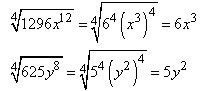Then a = 6x3b = 5y2, there is a "minus" sign in the middle, and:

1296x12 – 4320x9y2 + 5400x6y4 – 3000x3y6 + 625y8 = (6x3 – 5y2)4

Don't let the Binomial Theorem scare you. It's just another formula to memorize. A really complicated and annoying formula, I'll grant you, but just a formula, nonetheless. Don't overthink the Theorem; there is nothing deep or meaningful here. Just memorize it, and move on.

122 videos|142 docs

,

,

,

,

,

,

,

,

,

,

,

,

,

,

,

,

,

,

,

,

,

,

,

,

;